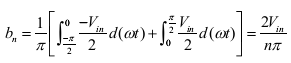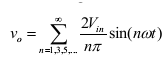Home > dc to ac inverter > half bridge dc ac inverter

# Half Bridge DC-AC Inverter

A single phase Half Bridge DC-AC inverter is shown in Figure below,

The analysis of the DC-AC inverters is done taking into accounts the following assumptions and conventions.
1) The current entering node a is considered to be positive.
2) The switches S1 and S2 are unidirectional, i.e. they conduct current in one direction.
3) The current through S1 is denoted as i1 and the current through S2 is i2.
The switching sequence is so design is shown in Figure below. Here,  switch S1 is on for the time duration  0 â‰¤ t â‰¤ T1 and the switch S2 is on for the time duration T1 â‰¤ t â‰¤ T2. When switch S1 is turned on, the instantaneous voltage across the load is  Î½ o = Vin/ 2
When the switch S2 is only turned on, the voltage across the load is
Î½ o = Ì¶ Vin/ 2.

The r.m.s value of output voltage Î½ o is given by,

The instantaneous output voltage Î½ o is rectangular in shape. The instantaneous value of Î½ o can be expressed in Fourier series as,

Due to the quarter wave symmetry along the time axis , the values of a0 and an are zero. The value of bn is given by,Substituting the value of bn from above equation 5, we getThe current through the resistor ( iL ) is given by,